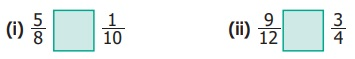Home | | Maths 6th Std | Ratio and Proportion

# Ratio and Proportion

Learning Objectives ● To understand the concept of ratio. ● To use ratio notation and simplify ratios. ● To divide a quantity into two parts in a given ratio. ● To recognise the relationship between ratio and proportion. ● To use the unitary method and solve simple ratio problems.

Chapter 3

RATIO AND PROPORTIONLearning Objectives

To understand the concept of ratio.

To use ratio notation and simplify ratios.

To divide a quantity into two parts in a given ratio.

To recognise the relationship between ratio and proportion.

To use the unitary method and solve simple ratio problems.

Recap

1. Which of the following fractions is not a proper fraction?

(a) 1/3

(b) 2/3

(c) 5/10

(d) 10 /5

2. The equivalent fraction of 17 is _________.

(a) 2/15

(b) 1/49

(c) 7/49

(d) 100 /7

3. Write > ,< or = in the box.(i) 5/8 > 1/10

(ii) 9 /12 = 3/4

4. Arrange these fractions from the least to the greatest : 1/2 , 1/4 , 6/8 , 1/8

Solution:

By taking LCM of denominators 2, 4, 8, 8

L.C.M = 8

Now 1/2 × 4/4 = 4/8; [1 × 2] / [4 × 2] = 2/8

LemLCM = 2 × 2 × 2 = 8       4/8, 2/8, 6/8, 1/8

Least to greatest = = 1/8, 2/8, 4/8, 6/8 = 1/8, 1/4, 1/2, 6/8

5. Anban says that 2/6 th of the group of triangles given below are blue. Is he correct?Answer: Wrong. 4/6 are blue, 2/6 are green

6. Joseph has a flower garden. Draw a picture which shows that 2/10 th of the flowers are red and the rest of them are yellow.

Solution:2/10 = Red and 8/10 = Yellow

7. Malarkodi has 10 oranges. If she ate 4 oranges, what fraction of oranges was not eaten by her?

Solution:

Total Oranges =10

Malarkodi ate = 4

Balance = 6 (10 − 4)

Fraction of oranges not eaten by her = 6/10

8. After sowing seeds on day one, Muthu observes the growth of two plants and records it. In 10 days, if the first plant grew 1/4 th of an inch and the second plant grew 3/8 th of an inch, then which plant grew more?

Solution: height of plant −I = 1/4 inch

height of plant − II = 3/8 inch

make them equal denominator

1/4 × 2/2 = 2/8

Now Plant –I = 2 / 8

Plant −II = 2/8

2/8 > 2/8 So plant − II grew more

Introduction

In our daily life, we handle lots of situations where we compare quantities. Comparison of our heights, weights, marks secured in examinations, speeds of vehicles, distances travelled, auto fare to taxi fare, bank balances at different periods of time and many more things are done. Comparison is usually between quantities of the same kind and not of different kind. It will not be meaningful to compare the height of a person with the age of another person. Also, we need a standard measure for comparison.

This sort of comparison by expressing one quantity as the number of times the other is called a ‘Ratio’.

MATHEMATICS ALIVE – RATIO IN REAL LIFETags : Term 1 Chapter 3 | 6th Maths , 6th Maths : Term 1 Unit 3 : Ratio and Proportion
Study Material, Lecturing Notes, Assignment, Reference, Wiki description explanation, brief detail
6th Maths : Term 1 Unit 3 : Ratio and Proportion : Ratio and Proportion | Term 1 Chapter 3 | 6th Maths